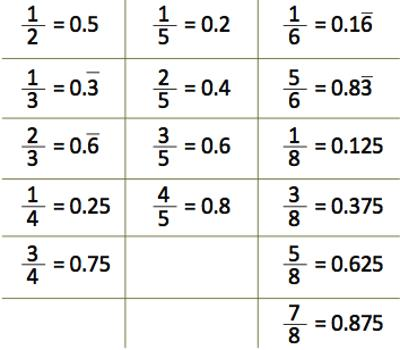Ordering Decimal Numbers

Decimal numbers have a whole number part and a decimal part. The decimal of a number represents the fractional part of the number. Decimal numbers can also be compared and ordered like integers. Ordering can be done from least decimal number in a given set of numbers to the greatest decimal number, and it will be known as ascending order of decimal numbers.

Numbers have an order or arrangement. The number two is between one and three. Three or more numbers can be placed in order. A number may come before the other numbers or it may come between them or after them.

Ordering decimals can be tricky. Because often we look at 0.42 and 0.402 and say that 0.402 must be bigger because there are more digits.

• Example: If we start with the numbers 4.3 and 8.78, the number 5.2764 would come between them, the number 9.1 would come after them and the number 2 would come before both of them.
• Example: If we start with the numbers 4.3 and 4.78, the number 4.2764 would come before both of them, the number 4.9 would come after them and the number 4.5232 would come between them.

The order may be ascending (getting larger in value) or descending (becoming smaller in value).Elaboration:

Decimals show values that are less than one. They use a continuation of the place values that show whole numbers. Each place value is ten times less as you move to the right. This means that the first place to the right of the decimal point (the decimal point separates the whole units from the decimal parts) is tenths, the next is hundredths, and then thousandths.

The arrangement from highest number to the least number is known as the descending order. To compare and order decimal numbers the whole number part and the decimal part are taken separately. If the whole number part is higher in one number than another then the value of that number will definitely be higher only. If in two decimal numbers whole number values are same then the decimal values are needed to be compared.

It can be helpful to visualize decimals using place value blocks. We can show one whole unit as a single cube which can be divided into ten equal parts (tenths) which can be divided into a further ten equal parts (hundredths) which can be further divided into ten equal parts (thousandths).

Real life Example:

The Glosser Family drove to a gasoline station in their neighborhood. The station has three gas pumps, each marked in price per gallon. Which pump has the lowest price per gallon? Which pump has the highest price per gallon?

\$1.79,  \$1.96,  \$1.61

Analysis: We know that 1.79 < 1.96 and that 1.79 > 1.61.

Answer: The pump marked \$1.61 has the lowest price per gallon. The pump marked \$1.96 has the highest price per gallon.

In the example above, we ordered three decimal numbers from least to greatest by comparing them two at a time.

Information Source: